### ReviewEcon.com

AP, IB, and College Microeconomic and Macroeconomic Principles

# Everything You Need To Know About Elasticity Coefficients

Updated 9/1/2023 Jacob Reed
The 2015 and 2016 Microeconomics exams both had FRQ’s focused on elasticity and calculating elasticity coefficients. Some of my own students felt inadequately prepared to tackle these tough questions.In an attempt to make sure that doesn’t happen again, I have created the elasticity review below along with a 15 question Elasticity Coefficient Calculations and Intepretations activity to test your skills. With a little luck it will provide you with everything you need to know about elasticity so you don’t get caught off guard when the AP Exam rolls around in May.

The Basics and Price Elasticity of Demand

Elasticity is the degree to which one change causes another change. Since price elasticity of demand is the simplest to understand, we’ll start with that. We know when prices increase, quantity demanded decreases. Elasticity tells us how much quantity demanded decreases by.

When a large change in price causes a small change in quantity demanded, the demand curve is relatively INelastic. That is, consumers are relatively INsensitive to the price change. Relatively inelastic demand curves tend to be more vertical than horizontal. If consumers demand the same quantity of a good regardless of the price, the demand curve is perfectly inelastic; consumers are perfectly INsensitive to the price change. Perfectly inelastic demand curves are vertical.

Goods that have inelastic demand curves tend to be:

• Products that are necessities.
• Products where there are few substitutes.
• Products that are relatively inexpensive.
When products have inelastic price elasticity of demand in the short run, there is a tendancy for these products to see large price increases over time. When that happens a demand curve will become more elastic in the long run. That is because substitutes will be introduced in the long run, allowing customers to become more price sensitive. For example, gasoline has inelastic demand in the short run, but in the long run demand for gasoline has become more elastic because high gas prices have led to substitutes. Today we have hybrid cars, electric cars, ethanol, and bio-fuels. All of these substitues, have increases the long-run price elasticity of the demand for gasoline.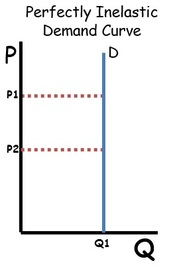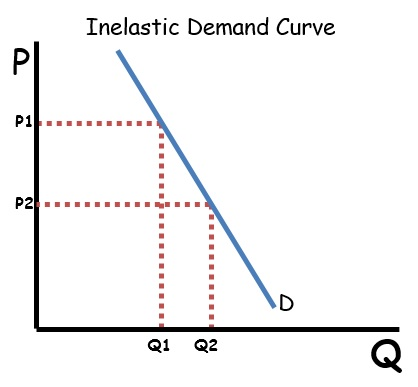When a small change in price causes a large change in quantity demanded, the demand curve is relatively Elastic. That is, consumers are Especially sensitive to the price change. Relatively elastic demand curves tend to be more horizontal than vertical. If consumers will demand any quantity at one maximum price, the demand curve is perfectly elastic; consumers are perfectly sensitive to the price change. Perfectly elastic demand curves are horizontal.

Goods that have elastic demand curves tend to be:

• Products that are not a necessity.
• Products where there are many substitutes.
• Products that are relatively expensive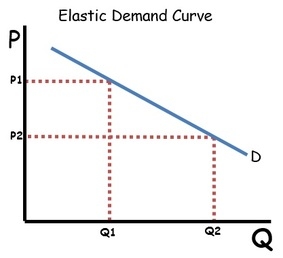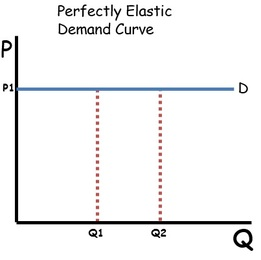There is another type of elasticity and it is called unit elastic. A unit elastic demand curve will have price increases cause proportional decreases in quantity demanded.

Note: Looking at the shape of a demand curve is not a sure way to determine elasticity. A linear demand curve’s elasticity decreases as quantities increase (see TR test below)

Total Revenue Test

When it comes to the price elasticity of demand, the simplest ways to determine elasticity is the total revenue (TR) test. The formula for total revenue is P x Q. On a demand curve, quantities fall as prices rise and quantities rise as prices fall. If price rises and TR increases (P and TR are going in the same direction), the demand curve is inelastic. If price falls and TR decreases (again P and TR are going in the same direction, the demand curve is also inelastic. If price rises and TR falls (P and TR are going in opposite directions, the demand curve is elastic. If the price falls and TR rises (again PR and TR are going in opposite directions, the demand curve is also elastic. If price changes do not change TR the demand curve is unit elastic (an increase or decrease in price keeps TR the same).

A straight line demand curve will have an elastic portion at the top, an inelastic portion on the bottom and a unit elastic point in the middle. If there is a Marginal Revenue curve on the graph (you will see that when learning about monopolies and  monopolistically competitive firms) it can help you determine elasticity. Decreasing price increases total revenue as quantity increases as long marginal revenue is greater than zero. The Unit Elastic portion of the demand curve is where the MR=0. Then decreasing price decreases total revenue since the marginal revenue is negative. In this range, the demand curve is inelastic.

Note: THE TOTAL REVENUE TEST ONLY APPLIES TO PRICE ELASTICITY OF DEMAND.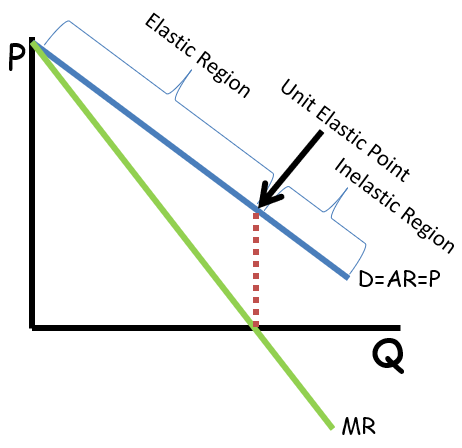Elasticity Coefficient

Another way to determine elasticity is to calculate the coefficient. A coefficient tells us the proportions at which a change in price changes quantity. A coefficient of -2 for example tells us that a price increase of a given percentage will cause twice as much decrease in quantity. A coefficient of -0.5, on the other hand, will cause a decrease in quantity demanded half (since 0.5 is 1/2) the percentage of the price increase. The basic formula for calculating a coefficient is the %∆Q/%∆P (∆ means change). After calculating the coefficient, the absolute value (meaning positive or negative doesn’t matter) can be used to determine the elasticity. Elasticity values are as follows:

• Absolute value of coefficient = 0: perfectly inelastic
• Absolute value of coefficient <1 (but not zero): relatively inelastic
• Absolute value of coefficient = 1: unit elastic
• Absolute value of coefficient >1 (but not ∞ or undefined): relatively elastic
• Absolute value of coefficient = ∞ or undefined: perfectly elastic.

Note: These are the elasticity values for all types of elasticity.

Elasticity Coefficient percentage change formulas: End Point Method

The end point method of calculating percentage change is the easiest and most straight forward. It has also been (so far) all that is needed to calculate coefficients on the released Advanced Placement Microeconomics Exams. The problem with this method is that it is not as precise as the mid-point formula and the direction of change can cause you problems.

Example: If the quantity increase from 100 to 150 that is an increase of 50% because (150 – 100)/100 x 100 = 50%. But if you go the opposite direction (from 150 to 100) you will get a change of 33 ⅓.% This difference will mean you get 2 different coefficients depending on which numbers you use as the new and old. The trick is to follow the direction in the question. So if quantity increase from 100 to 150 when price decreases from \$100 to \$75, the percentage change of quantity will be 50% and the percentage change of price will be -25%. And %∆Q/%∆P=50%/-25%=-2 so this demand curve is price elastic.

Elasticity Coefficient percentage change formulas: Midpoint Method

The midpoint method of calculating percentage change eliminates the directional issues associated with calculating percentage change. That is because it takes an average middle point between the new and old prices/quantities, to calculate the percent change.

The downside to this the math with this method is often more difficult. The method you prefer is really up to you. But choose wisely.

Price elasticity

As mentioned above, %∆Q/%∆P will give you a price elasticity coefficient. Demand curves have a negative price elasticity coefficient due to the demand curve’s inverse relationship between price and quantity. Supply curves have a positive price elasticity coefficient due to the direct relationship between price and quantity. Price elasticity of demand is determined by the number of substitutes, how necessary the item is, and how much of a consumer’s income it takes to pay for the product. Price elasticity of supply is determined by the prices of alternative inputs, availablility of inputs, time necessary to produce the product, etc.

Income elasticity

Income elasticity is about how much a change in consumer income causes a change in quantity demanded. Normal goods (most goods fall into this category) are goods that consumers buy more of when their incomes rise, and less of when their incomes fall. Inferior goods are goods like one-ply toilet paper, top ramen, or generic brand products. When consumers’ incomes rise, consumers buy less of these goods, and when incomes fall, they buy more.

One of the non-price determinants of demand is changes in income. Income elasticity tells us how much a change in income will shift the demand for a good or service. The formula for income elasticity is %∆Q/%∆Income. Normal goods have a positive income elasticity coefficient since increases in incomes cause increases in the demand for normal goods. Inferior goods have a negative income elasticity coefficient. This is because increases in incomes cause decreases in the demand for inferior goods.

Cross-Price elasticity

Cross-price elasticity is about substitutes and complements. Substitutes are goods that can be used in place of each other; like butter and margarine, or jam and jelly. When the price of one increases, the demand for the other also increases. Complements are goods that are used together; like bread and butter or tooth brushes and toothpaste. When the price of one increases, demand for the other decreases. The cross-price elasticity tells us how much a change in the price of one impacts the demand for the other.

When there is a change in the price of substitute or complement, the demand for the good in question will change. The formula for cross-price elasticity is %∆Q/%∆P (P is the price of the other good). Substitute goods will have a positive coefficient because an increase in the price of a substitute will cause an increase in the demand for the good in question. Complementary goods will have a negative coefficient because an increase in the price of a complement will cause a decrease in the demand for the good in question.

Multiple Choice Connections:
2012 Released AP Microeconomics Exam Questions: 33, 34

Up Next:
Review Game: Elasticity Coefficients Calcualation and Interpretation
Content Review Page: Excise Taxes

Other recommended resourcesACDC Video (Elasticity of Demand, Total Revenue), ACDC Video (Income, Cross-Price)